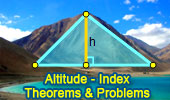# Altitude of a Triangle, Theorems and Problems - Table of Content 4Proposed Problem 135. Orthic Triangle, Altitudes, Perpendicular, Parallel

Proposed Problem 134. Orthic Triangle, Altitudes, Angle Bisectors, Orthocenter, Incenter.

Proposed Problem 91. Similar Triangles, Altitudes, Parallel.

Taylor Circle Theorem Feet of each altitude.
Using TracenPoche Dynamic Software
Step-by-Step construction, Manipulation, and animation.
Henry Martyn Taylor and the blind student of mathematics

Proposed Problem 79: Triangle. Similarity, Altitudes, Orthocenter, Incircles, Inradii.

Feuerbach Points and Nine-Point Circle with interactive animation, manipulation, and step-by-step construction.

Nine-Point Center, Nine-Point Circle, Euler Line.
Interactive illustration.
Altitudes, Orthocenter.

Geometry Problem 800: de Gua's Theorem
Pythagorean theorem in 3-D, Tetrahedron, Cubic Vertex, Triangular Pyramid, Apex, Height, Right Triangle Area, Base Area, Projected Area.

Triangle and Squares 22
Altitudes

Proposed Problem 51: Fagnano's Problem
Inscribed Triangle with the Minimum Perimeter.
Altitudes.

Right Triangles, Formulas and Facts
Pythagorean theorem, Similarity, Geometric mean, hypotenuse, catheti (legs), semiperimeter, altitude, median, similar shapes areas, centers, orthocenter, incenter, centroid, circumcenter, radius, inradius, circumradius, exradius, circles, incircle, circumcircle, excircle.

Triangle and Squares 20
Altitude.

Proposed Problem 49. Right triangle, Altitude, Angles.

Go to page: Previous | 1 | 2 | 3 | 4 | 5 | Next

 Home | Search | Geometry | Post a comment | Email | By Antonio Gutierrez